Next: Half Integral Weight Modular Up: Computing with Classical Modular Previous: Weight   Contents

# A Problem About Bernoulli Numbers

This section was written by Ralph Greenberg.

Definition 4.4.1 (Irregular Prime)   A prime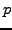is said to be irregular ifdivides the numerator of a Bernoulli number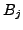, where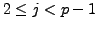and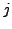is even. (For odd, one has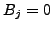.)

The index of irregularity for a primeis the number of such.'s There is considerable numerical data concerning the statistics of irregular primes - the proportion of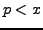which are irregular or which have a certain index of irregularity. (See Irregular primes and cyclotomic invariants to four million, Buhler et al., in Math. of Comp., vol. 61, (1993), 151-153.)

Let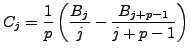for eachas above. According to the Kummer congruences,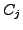is a-integer, i.e., its denominator is not divisible by. But its numerator could be divisible by. This happens for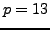and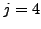.

Problem 4.4.2   Obtain numerical data for the divisibility of the numerator ofby a primeanalogous to that for the's.

Motivation: It would be interesting to find an example of a primeand an index(with,even) such thatdivides the numerator of bothand. Then the-adic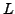-function for a certain even character of conductor(namely, the-adic valued character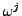, where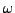is the character characterized by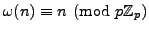for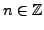) would have at least two zeros. No such example exists for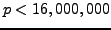. The-adic-functions for those primes have at most one zero. If the statistics for the's are similar to those for the's, then a probabilistic argument would suggest that examples should exist.

Problem 4.4.3   Computation offor a specificis very efficient in PARI, hence in SAGE via the command bernoulli. Methods for computation of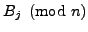for a large range ofare described in Irregular primes and cyclotomic invariants to four million, Buhler et al. Implement the method of Buhler et al. in SAGE.Next: Half Integral Weight Modular Up: Computing with Classical Modular Previous: Weight   Contents
William Stein 2006-10-20